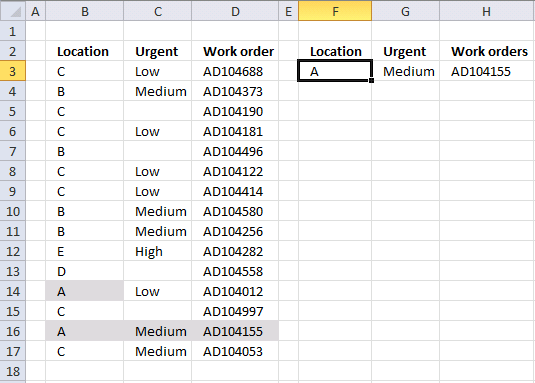Author: Oscar Cronquist Article last updated on October 12, 2018

This animated picture shows you the most urgent work orders for a location. Type a location in cell F3 and the formula in cell G3 extracts the most urgent value for that particular location. The values in column C are 0 (blank), Low, Medium and High.I have added conditional formatting to the table so you can quickly verify that the formulas in cell G3 and H3 are correct.

Array formula in cell H3:

=INDEX({""; "Low"; "Medium"; "High"}, MAX(IF(F3=B3:B17, MATCH(\$C\$3:\$C\$17, {0; "Low"; "Medium"; "High"}, 0), "")))

Array formula in cell H3 can be found in this post: How to return multiple values using vlookup

### Explaining the array formula in cell H3

Cell value in F3 is A in the calculations below.

Step 1 - Calculate the relative positions of values in column C with these values {0; "Low"; "Medium"; "High"}

MATCH(\$C\$3:\$C\$17, {0; "Low"; "Medium"; "High"}, 0) returns {2; 3; 1; 2; 1; 2; 2; 3; 3; 4; 1; 2; 1; 3; 3}

Step 2 - Extract values matching cell F3

IF(F3=B3:B17, MATCH(\$C\$3:\$C\$17, {0; "Low"; "Medium"; "High"}, 0), "") returns {""; ""; ""; ""; ""; ""; ""; ""; ""; ""; ""; 2;""; 3;""}

Step 3 - Find the largest value

MAX(IF(F3=B3:B17, MATCH(\$C\$3:\$C\$17, {0; "Low"; "Medium"; "High"}, 0), "")) returns 3.

Step 4 - Return urgent value

=INDEX({""; "Low"; "Medium"; "High"}, MAX(IF(F3=B3:B17, MATCH(\$C\$3:\$C\$17, {0; "Low"; "Medium"; "High"}, 0), ""))) returns Medium in cell G3.

The MATCH and MAX function returns the most urgent value in column C, the order of values in the array determines the importance. {0;"Low";"Medium","High"}. For example, "Medium" is more urgent than "Low". The MATCH function matches one set of values (column C) with another set of values {0;"Low";"Medium","High"}.

That makes the MATCH function really amazing, if you combine multiple MATCH functions you can work with related tables:

Find most urgent work orders.xlsx

### Functions in this post:

INDEX(array,row_num,[column_num])
Returns a value or reference of the cell at the intersection of a particular row and column, in a given range

MAX(number1, number2)
Returns the largest value in a set of values

MATCH(lookup_value, lookup_array, [match_type]
Returns the relative position of an item in an array that matches a specified value

IF(logical_test, [value_if_true], [value_if_false])
Checks whether a condition is met, and returns one value if TRUE, and another value if FALSE